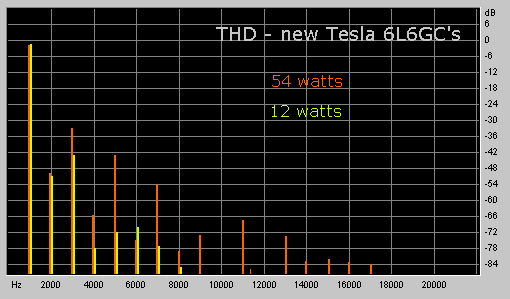You have probably seen "Total Harmonic Distortion" figures, expressed in percent, applied as one measure of quality of audio gear. What does this mean, and how is it measured?

 Harmonic Voltage Power % Distortion Fundamental 4.0 volts 2 watts 2nd Harm. 0.3 volts .01125 W 0.56225 % 3rd Harm. 0.5 volts .03125 W 1.5625 % Total .0425 W 2.12475 %

"Hey, neat," you may be saying. "Only 2.12475%!" But we're not there yet; 2.12475% is another wrong answer. While this apparent THD figure is, indeed, representative of the total power of the harmonic content, we still have to convert this power to an equivalent RMS voltage in order to come up with a THD figure according to standard methodology.

So the correct answer is obtained by taking total harmonic power (0.0425 watts) and calculating the equivalent RMS voltage, given by the square root of power times output impedance, or about 0.583 volts in the example. i.e. SQRT(0.0425 watts * 8 ohms). So the THD value, as specified would actually be 100 times 0.583 volts divided by 4 volts = about 14.6% (correct answer).

To generalize this as an equation,

 THD(%) = 100 * SQRT[(P2 + P3 + P4 + ... + Pn) * Zout] / Vt

where TDH(%) is total harmonic distortion, P represents the power of each harmonic, Zout equals the load impedance, and Vt is the total RMS output voltage (containing both the fundamental and the harmonic terms).

Another way of getting the correct answer would be to take the square root of the sum of the squares of harmonic component voltages. This is because power is proportional to the square of the voltage. Let's try it. SQRT(0.32 + 0.52) = SQRT(.09 + .25) = SQRT(0.34) = 0.583. Multiply by 100 (to get percent) and divide by 4 (fundamental voltage) gives the same correct result, approx. 14.6%.

In equation form,

 THD(%) = 100 * SQRT[(V22 + V32 + V42 + ... + Vn2)] / Vt

where TDH(%) is total harmonic distortion, V represents the RMS voltage of each harmonic, and Vt is the total RMS output voltage.
Note that Zout is not present in this variant of the equation;
since P = V2/Z, the impedance terms cancel.

 Working from a Spectrogram The above approach is quite workable if you have, say, a tunable filter and RMS-calibrated voltmeter or oscilloscope with which you can get the harmonic distortion voltages. However, in this day and age, it's usually easier, cheaper and possibly more accurate to use an FFT spectrograph program to analyse your output signal. A typical output graph (describing the harmonic distortion behaviour of my RA-100 amplifier with new Tesla/JJ tubes) is shown below:I find this visual approach much more informative than a single THD number, since the relative strength of each harmonic is clearly illustrated; also, other relationships become readily apparent, such as (in this case) the attenuation of even harmonics because of the circuit's push-pull design. But for the sake of comparison (e.g. with similar designs) how would you derive the TDH% figure from the graph? Doing it by hand would be even more tedious than the earlier examples, since relative output is generally shown in decibels. So for each harmonic, you would have to compute first the power represented by each component, then derive the voltage, and finally do the "square root of the sum of the squares" thing to come up with a single THD figure. So why not put your computer to work for this also? I've worked up a simple spreadsheet, which you can download either in Excel (thd.xls) or Lotus 1-2-3 (thd.wks) format. When you open the spreadsheet, you'll see this:Both "instances" operate independantly, so you can easily do side-by-side comparisons of two sets of data (as, in this case, between the RA-100 at 12 watts and at 54 watts). To use this, first normalise your wave so that the peaks reach 100 percent, then do a FFT spectrum analysis. The result will be a graph similar to those shown here. Finally, read off the decibel values of each harmonic, and enter them into the appropriate cells in the "dB"column of the spreadsheet. For values that are "off the graph" enter some absurdly low dB value (e.g. -100) and the resultant computation for that harmonic will be negligible (unless you have a really really good amp!) ;-) As an aside, it should be noted that your graphs will normally show the fundamental at slightly less than 0 dB, even if you first normalised your output wave to 100%. This is not an error in your program (for instance "CoolEdit"), it is due to the fact that the harmonic content effectively subtracts from the fundamental power. You can have a look at the formulae in the "Power" columns and "Totals" rows if you want to understand what's going on here. I hope that some of you will find this useful.

Back to Fred's Vacuum